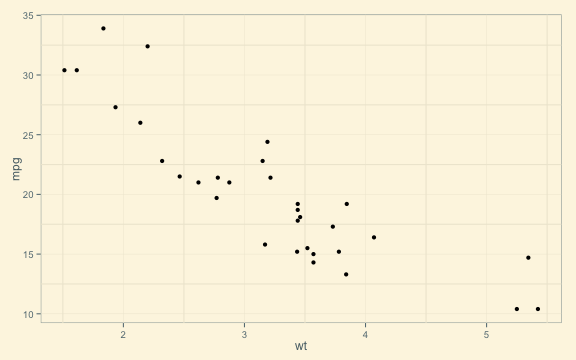# Slidify

## Reproducible HTML5 Slides

Ramnath Vaidyanathan
Assistant Professor, McGill

1. Mathjax
2. Layouts
3. Backgrounds
4. Widgets
5. Overlays
7. Embeds

## Nested Lists

• Thing 1

• Point 1
• Point 2
• Thing 2

• Point 3
• Point 4

Tab1

Tab2

Tab3

This slide shows a Setext style header.

## Tables

Mardown tables contain --- and should not be interpreted as separator.

Column X Column Y
Row 1 Row 1
Row 2 Row 2

## Animated List, Ordered

This list should be animated

1. Point 1
2. Point 2
3. Point 3

## Animated List, Unordered

This list should be animated

• Point 1
• Point 2
• Point 3

## Mathjax

\begin{aligned} \nabla \times \vec{\mathbf{B}} -\, \frac1c\, \frac{\partial\vec{\mathbf{E}}}{\partial t} & = \frac{4\pi}{c}\vec{\mathbf{j}} \\ \nabla \cdot \vec{\mathbf{E}} & = 4 \pi \rho \\ \nabla \times \vec{\mathbf{E}}\, +\, \frac1c\, \frac{\partial\vec{\mathbf{B}}}{\partial t} & = \vec{\mathbf{0}} \\ \nabla \cdot \vec{\mathbf{B}} & = 0 \end{aligned}
$\mathbf{V}_1 \times \mathbf{V}_2 = \begin{vmatrix} \mathbf{i} & \mathbf{j} & \mathbf{k} \\ \frac{\partial X}{\partial u} & \frac{\partial Y}{\partial u} & 0 \\ \frac{\partial X}{\partial v} & \frac{\partial Y}{\partial v} & 0 \end{vmatrix}$

This is a slide with a two column layout.

Column 1

Column 2

## Background Color

This slide should have a subtle gray background.

## Background Image

This slide should have a background image.

## Widgets: jQuery-Quiz

This is a multiple choice question

1. Choice 1
2. Choice 2
3. Choice 3 (correct)
4. Choice 4

This is a hint

This is the explanation

## Widgets: Bootstrap

Blocks

This is an alert info block which should render in blue

Tooltips

This is to check out tooltips in bootstrap you probably

Popover

cd data

## Highlighter: Highlight JS

We can make this function more robust by adding a simple error checking statement to prevent illegal parameters from generating invalid results.

qpareto <- function(p, scale, loc) {
if ((scale <= 0) | (loc <= 0)) {
stop("'qpareto' parameters must be greater than zero.")
}
q <- loc * (1 - p)^(-1/scale)
return(q)
}


Entering a negative parameter now raises an exception, instead of an invalid result that could silently corrupt subsequent results.

qpareto(0.4, 5, -1)

## Error: 'qpareto' parameters must be greater than zero.


We could improve this further by checking to ensure that p is a valid probability.

## Check Chunk Execution

library(ggplot2)
library(ggthemes)

## Warning: package 'ggthemes' was built under R version 2.15.3

qplot(wt, mpg, data = mtcars) + theme_solarized()This is a shout

I am checking key, key